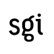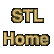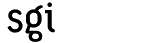# Function Objects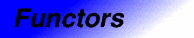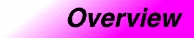Category: functors Component type: overview

### Summary

A Function Object, or Functor (the two terms are synonymous) is simply any object that can be called as if it is a function. An ordinary function is a function object, and so is a function pointer; more generally, so is an object of a class that defines operator().

### Description

The basic function object concepts are Generator, Unary Function, and Binary Function: these describe, respectively, objects that can be called as f(), f(x), and f(x,y). (This list could obviously be extended to ternary function and beyond, but, in practice, no STL algorithms require function objects of more than two arguments.) All other function object concepts defined by the STL are refinements of these three.

Function objects that return bool are an important special case. A Unary Function whose return type is bool is called a Predicate, and a Binary Function whose return type is bool is called a Binary Predicate.

There is an important distinction, but a somewhat subtle one, between function objects and adaptable function objects.  In general, a function object has restrictions on the type of its argument. The type restrictions need not be simple, though: operator() may be overloaded, or may be a member template, or both. Similarly, there need be no way for a program to determine what those restrictions are. An adaptable function object, however, does specify what the argument and return types are, and provides nested typedefs so that those types can be named and used in programs. If a type F0 is a model of Adaptable Generator, then it must define F0::result_type. Similarly, if F1 is a model of Adaptable Unary Function then it must define F1::argument_type and F1::result_type, and if F2 is a model of Adaptable Binary Function then it must define F2::first_argument_type, F2::second_argument_type, and F2::result_type. The STL provides base classes unary_function and binary_function to simplify the definition of Adaptable Unary Functions and Adaptable Binary Functions. 

Adaptable function objects are important because they can be used by function object adaptors: function objects that transform or manipulate other function objects. The STL provides many different function object adaptors, including unary_negate (which returns the logical complement of the value returned by a particular AdaptablePredicate), and unary_compose and binary_compose, which perform composition of function object.

Finally, the STL includes many different predefined function objects, including arithmetic operations (plus, minus, multiplies, divides, modulus, and negate), comparisons (equal_to, not_equal_to greater, less, greater_equal, and less_equal), and logical operations (logical_and, logical_or, and logical_not). It is possible to perform very sophisticated operations without actually writing a new function object, simply by combining predefined function objects and function object adaptors.

### Examples

Fill a vector with random numbers. In this example, the function object is simply a function pointer.
```    vector<int> V(100);
generate(V.begin(), V.end(), rand);
```

Sort a vector of double by magnitude, i.e. ignoring the elements' signs. In this example, the function object is an object of a user-defined class.

```    struct less_mag : public binary_function<double, double, bool> {
bool operator()(double x, double y) { return fabs(x) < fabs(y); }
};

vector<double> V;
...
sort(V.begin(), V.end(), less_mag());
```

Find the sum of elements in a vector. In this example, the function object is of a user-defined class that has local state.

```    struct adder : public unary_function<double, void>
{
double sum;
void operator()(double x) { sum += x; }
};

vector<double> V;
...
cout << "The sum is " << result.sum << endl;
```

Remove all elements from a list that are greater than 100 and less than 1000.

```    list<int> L;
...
list<int>::iterator new_end =
remove_if(L.begin(), L.end(),
compose2(logical_and<bool>(),
bind2nd(greater<int>(), 100),
bind2nd(less<int>(), 1000)));
L.erase(new_end, L.end());
```

### Notes

 The reason for the name "adaptable function object" is that adaptable function objects may be used by function object adaptors.

 The unary_function and binary_function bases are similar to the input_iterator, output_iterator, forward_iterator, bidirectional_iterator, and random_access_iterator bases: they are completely empty, and serve only to provide type information.

 This is an example of how to use function objects; it is not the recommended way of calculating the sum of elements in a vector. The accumulate algorithm is a better way of calculating a sum.